• ## 傅里叶变换 二维离散傅里叶变换

万次阅读 热门讨论 2019-11-07 15:41:28
DFT:(Discrete Fourier Transform)离散傅里叶变换是傅里叶变换在时域和频域上都呈离散的形式，将信号的时域采样变换为其DTFT的频域采样。在形式上，变换两端（时域和频域上）的序列是有限长的，而实际上这两组序列...
1、介绍。

DFT:(Discrete Fourier Transform)离散傅里叶变换是傅里叶变换在时域和频域上都呈离散的形式，将信号的时域采样变换为其DTFT的频域采样。在形式上，变换两端（时域和频域上）的序列是有限长的，而实际上这两组序列都应当被认为是离散周期信号的主值序列。即使对有限长的离散信号作DFT，也应当将其看作其周期延拓的变换。

1）、欧拉公式：

，其中i是虚数，即i的平方为-1。

2）、二维离散傅里叶变换DFT公式：

N是二维数组的行数，M是二维数组的列数。u和v是转换后二维数组的位置，F(u,v)是转换后数组中相应位置的值。x和y是原二维数组的位置，f(x,y)是原数组中相应的值。

根据欧拉公式，也可以写成：

3）、二维离散傅里叶逆变换IDFT公式：

N是二维数组的行数，M是二维数组的列数。x和y是转换后二维数组的位置，f(x,y)是转换后数组中相应位置的值。u和v是原二维数组的位置，F(u,v)是原数组中相应的值。

根据欧拉公式，也可以写成：

2、生成随机的二维数组。

//f(x,y)=1+2x+8x^2+2y+3xy^2
private static double[][] getRandom2(int row, int column) {
double[][] arrays = new double[row][column];
for (int x = 0; x < row; x++) {
for (int y = 0; y < column; y++) {
double value = 1 + 2 * x + 8 * x * x + 2 * y + 3 * x * y * y;
arrays[x][y] = value;
}
}
return arrays;
}

3、复数类。参考文章高数 复数的四则运算

package com.zxj.reptile.utils.number;

public class Complex {
private double real;//实数
private double image;//虚数

public Complex() {
real = 0;
image = 0;
}

public Complex(double real, double image) {
this.real = real;
this.image = image;
}

//加：(a+bi)+(c+di)=(a+c)+(b+d)i
double real = complex.getReal();
double image = complex.getImage();
double newReal = this.real + real;
double newImage = this.image + image;
return new Complex(newReal, newImage);
}

//减：(a+bi)-(c+di)=(a-c)+(b-d)i
public Complex sub(Complex complex) {
double real = complex.getReal();
double image = complex.getImage();
double newReal = this.real - real;
double newImage = this.image - image;
return new Complex(newReal, newImage);
}

public Complex mul(Complex complex) {
double real = complex.getReal();
double image = complex.getImage();
double newReal = this.real * real - this.image * image;
double newImage = this.image * real + this.real * image;
return new Complex(newReal, newImage);
}

public Complex mul(double multiplier) {
return mul(new Complex(multiplier, 0));
}

public Complex div(Complex complex) {
double real = complex.getReal();
double image = complex.getImage();
double denominator = real * real + image * image;
double newReal = (this.real * real + this.image * image) / denominator;
double newImage = (this.image * real - this.real * image) / denominator;
return new Complex(newReal, newImage);
}

//欧拉公式 e^(ix)=cosx+isinx
public static Complex euler(double x) {
double newReal = Math.cos(x);
double newImage = Math.sin(x);
return new Complex(newReal, newImage);
}

public double getReal() {
return real;
}

public void setReal(double real) {
this.real = real;
}

public double getImage() {
return image;
}

public void setImage(double image) {
this.image = image;
}

@Override
public String toString() {
String str = "";
if (real != 0) {
str += real;
} else {
str += "0";
}
if (image < 0) {
str += image + "i";
} else if (image > 0) {
str += "+" + image + "i";
}
return str;
}
}


4、二维离散傅里叶变换DFT代码。

    public static Complex[][] getDft2(double[][] arrays) {
int M = arrays.length;
int N = arrays.length;
Complex[][] complexArrays = new Complex[M][N];
double flag = -2 * Math.PI;
for (int u = 0; u < M; u++) {
for (int v = 0; v < N; v++) {
//x-->M和y-->N的累加
Complex sum = new Complex();
for (int x = 0; x < M; x++) {
for (int y = 0; y < N; y++) {
//e^(-(2 * PI * (ux / M + vy / N)i)
double angle = flag * (u * x * 1.0 / M + v * y * 1.0 / N);
Complex complex = Complex.euler(angle);
//f(x) * e^(-(2 * PI * (ux / M + vy / N)i)
complex = complex.mul(arrays[x][y]);
}
}
complexArrays[u][v] = sum;
}
}
return complexArrays;
}

5、二维离散傅里叶逆变换IDFT代码。

    public static double[][] getInverseDft2(Complex[][] complexArrays) {
int M = complexArrays.length;
int N = complexArrays.length;
double[][] arrays = new double[M][N];
double flag = 2 * Math.PI;
for (int x = 0; x < M; x++) {
for (int y = 0; y < N; y++) {
//u-->M和v-->N的累加
Complex sum = new Complex();
for (int u = 0; u < M; u++) {
for (int v = 0; v < N; v++) {
//e^((2 * PI * (ux / M + vy / N)i)
double angle = flag * (u * x * 1.0 / M + v * y * 1.0 / N);
Complex complex = Complex.euler(angle);
//f(x) * e^((2 * PI * (ux / M + vy / N)i)
complex = complex.mul(complexArrays[u][v]);
}
}
//f(x) * e^((2 * PI * (ux / M + vy / N)i) / (M * N)
sum = sum.mul(1 * 1.0 / (N * M));
double realSum = sum.getReal();
arrays[x][y] = Math.round(realSum * 100) / 100.0;
}
}
return arrays;
}

6、因为java的Arrays类没有提供二维数组是否相等的比较方法，所以自己写一个比较二维数组是否相等的方法。

public static boolean equals(double[][] a, double[][] b) {
if (a == null || b == null || a.length != b.length) {
return false;
}
for (int i = 0; i < a.length; i++) {
if (!Arrays.equals(a[i], b[i])) {
return false;
}
}
return true;
}

7、流程。可以先将随机数组的大小设小点，然后将打印日志的代码开起来，这样子才会对流程有更多的了解。

    public static void main(String[] args) {
System.out.println("------开始------");
long time = System.currentTimeMillis();
// testDft();//一维离散傅里叶变换
testDft2(10, 10);//二维离散傅里叶变换
System.out.println("花费时间 ：" + (System.currentTimeMillis() - time));
System.out.println("------结束------");
}

private static void testDft2(int row, int column) {
System.out.println(String.format("二维数组共有%d行%d列", row, column));
//System.out.println("原数据: ");
double[][] arrays = getRandom2(row, column);
for (int x = 0; x < row; x++) {
for (int y = 0; y < column; y++) {
//System.out.print(arrays[x][y] + " ");
}
//System.out.println();
}
//System.out.println("离散傅里叶变换DFT: ");
Complex[][] dtfArrays = FourierUtils.getDft2(arrays);
for (int x = 0; x < row; x++) {
for (int y = 0; y < column; y++) {
//System.out.print(dtfArrays[x][y] + " ");
}
//System.out.println();
}
//System.out.println("逆离散傅里叶变换IDFT: ");
double[][] inverseDtfArrays = FourierUtils.getInverseDft2(dtfArrays);
for (int x = 0; x < row; x++) {
for (int y = 0; y < column; y++) {
//System.out.print(inverseDtfArrays[x][y] + " ");
}
//System.out.println();
}
System.out.print("二维离散傅里叶变换和逆离散傅里叶变换");
if (ArrayUtils.equals(arrays, inverseDtfArrays)) {
System.out.println("成功");
} else {
System.out.println("失败");
}
}

8、结果。可以将生成随机二维数组的大小进行改变，也可以对生成随机数据的函数进行改变。会发现原数组和经过离散傅里叶变换DFT及逆离散傅里叶变换IDFT之后的数组是一模一样的，就可以验证出算法和公式是对的。我将打印的日志和结果截图一并给出来。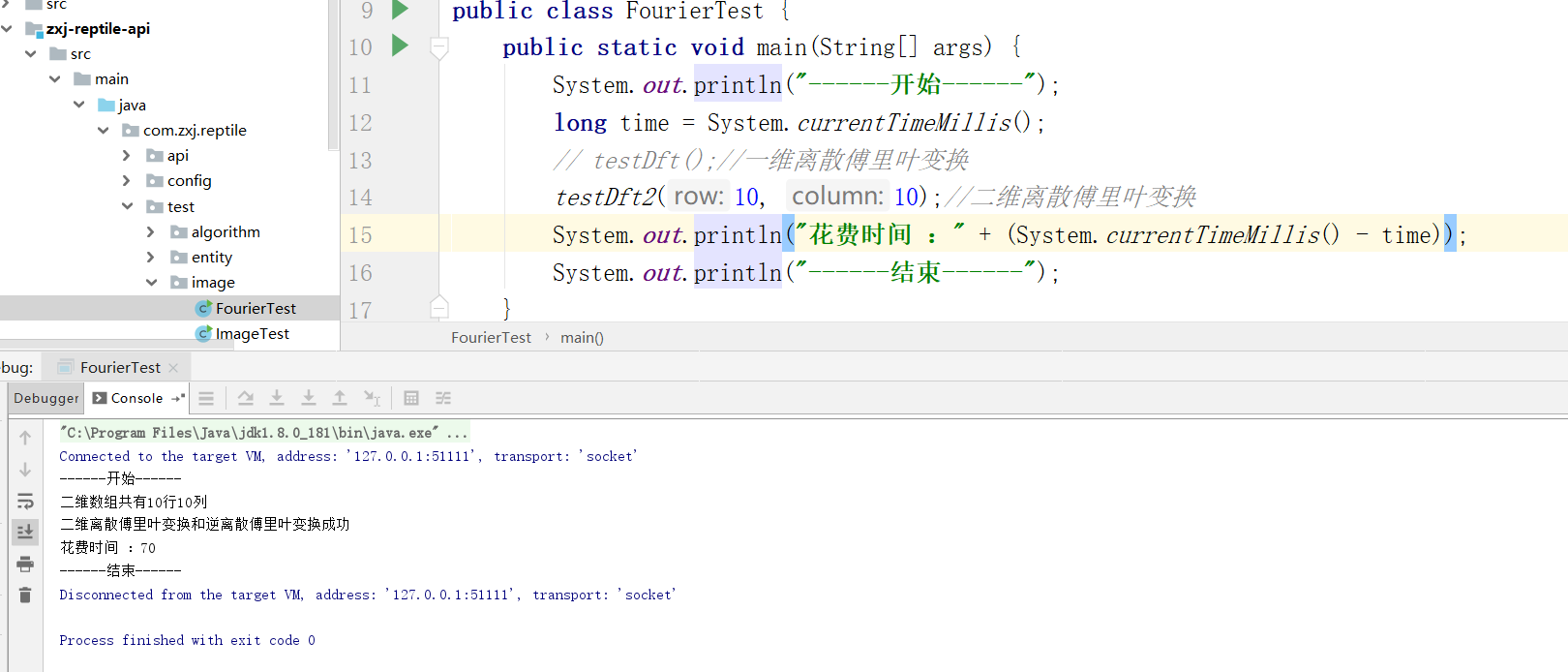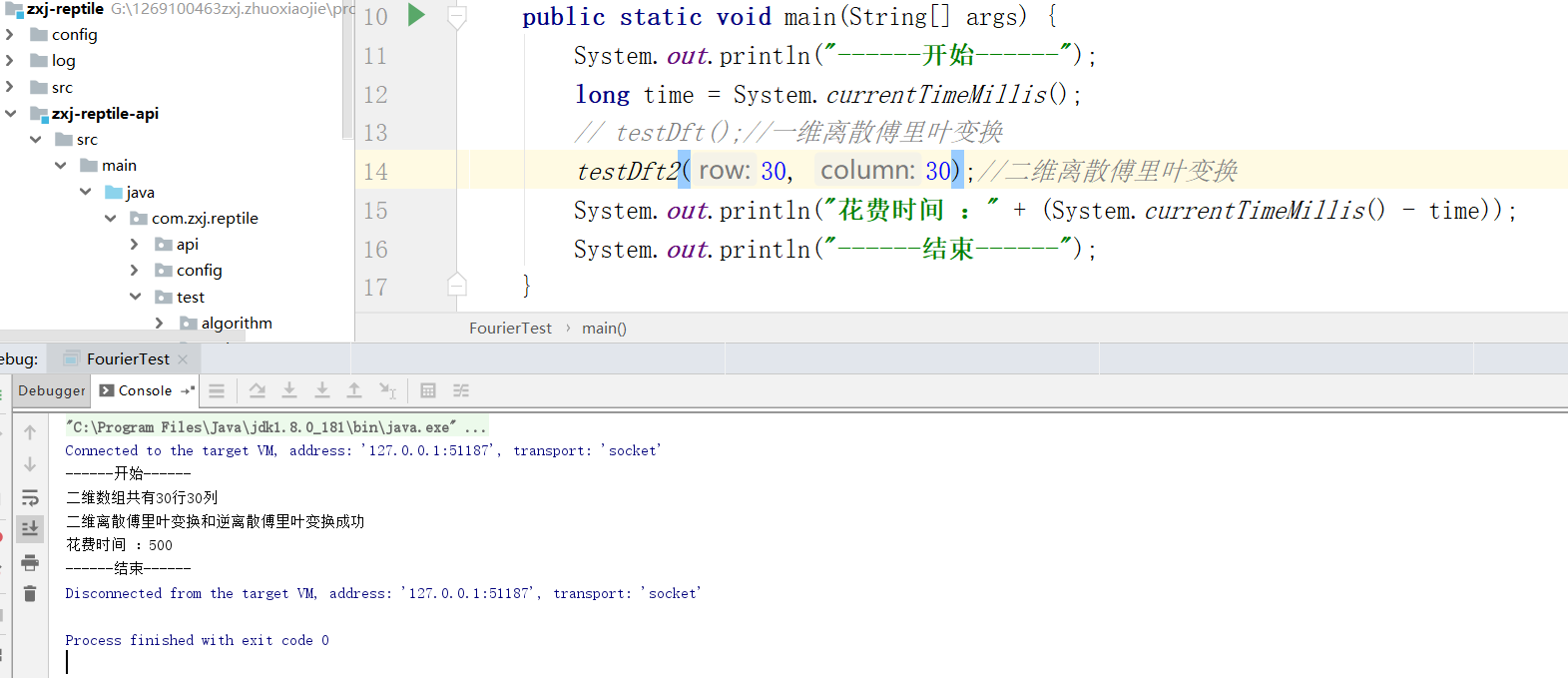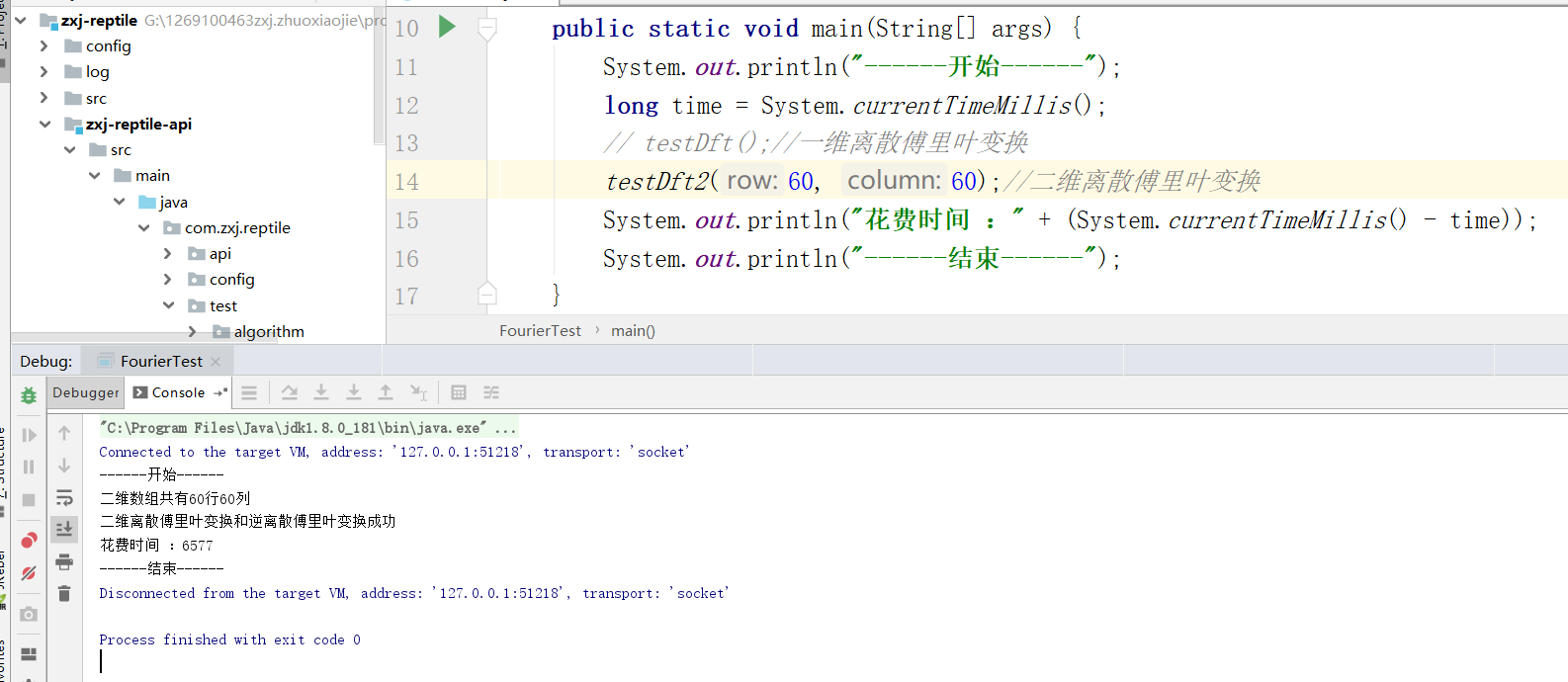9、结论。

二维离散傅里叶因为涉及到4个嵌套循环，时间复杂度十分的大，所以在实际应用场景上得不到使用，现在的照片小的差不多也要500*500的大小，但是二维离散傅里叶处理大小60*60的时候，就已经显得十分的吃力了。


展开全文二维DFT
• ## 傅里叶变换 一维离散傅里叶变换

万次阅读 热门讨论 2019-11-06 21:08:43
DFT:(Discrete Fourier Transform)离散傅里叶变换是傅里叶变换在时域和频域上都呈离散的形式，将信号的时域采样变换为其DTFT的频域采样。在形式上，变换两端（时域和频域上）的序列是有限长的，而实际上这两组序列...
1、介绍。

DFT:(Discrete Fourier Transform)离散傅里叶变换是傅里叶变换在时域和频域上都呈离散的形式，将信号的时域采样变换为其DTFT的频域采样。在形式上，变换两端（时域和频域上）的序列是有限长的，而实际上这两组序列都应当被认为是离散周期信号的主值序列。即使对有限长的离散信号作DFT，也应当将其看作其周期延拓的变换。实际应用的时候，都是使用快速傅里叶变换的，因为运算速度快。

1）、欧拉公式：

，其中i是虚数，即i的平方为-1。

2）、一维离散傅里叶变换DFT公式：

u是转换后一维数组的位置，F(u)是转换后数组中相应位置的值。x是原一维数组的位置，f(x)是原数组中相应的值。

，其中i是虚数。

一维DFT公式中的就是欧拉公式中的，而且，。

所以一维DFT公式又可以写成：。

3）、一维离散傅里叶逆变换IDFT公式：

x是转换后一维数组的位置，f(x)是转换后数组中相应位置的值。u是原一维数组的位置，F(u)是原数组中相应的值。

。

又可以写成：。

2、生成随机的一维数组。

    //f(x)=1+2x
private static double[] getRandom(int length) {
double[] array = new double[length];
for (int x = 0; x < length; x++) {
double value = 1 + 2 * x;
array[x] = value;
}
return array;
}

3、复数的类。参考文章高数 复数的四则运算

package com.zxj.reptile.utils.number;

public class Complex {
private double real;//实数
private double image;//虚数

public Complex() {
real = 0;
image = 0;
}

public Complex(double real, double image) {
this.real = real;
this.image = image;
}

//加：(a+bi)+(c+di)=(a+c)+(b+d)i
double real = complex.getReal();
double image = complex.getImage();
double newReal = this.real + real;
double newImage = this.image + image;
return new Complex(newReal, newImage);
}

//减：(a+bi)-(c+di)=(a-c)+(b-d)i
public Complex sub(Complex complex) {
double real = complex.getReal();
double image = complex.getImage();
double newReal = this.real - real;
double newImage = this.image - image;
return new Complex(newReal, newImage);
}

public Complex mul(Complex complex) {
double real = complex.getReal();
double image = complex.getImage();
double newReal = this.real * real - this.image * image;
double newImage = this.image * real + this.real * image;
return new Complex(newReal, newImage);
}

public Complex mul(double multiplier) {
return mul(new Complex(multiplier, 0));
}

public Complex div(Complex complex) {
double real = complex.getReal();
double image = complex.getImage();
double denominator = real * real + image * image;
double newReal = (this.real * real + this.image * image) / denominator;
double newImage = (this.image * real - this.real * image) / denominator;
return new Complex(newReal, newImage);
}

//欧拉公式 e^(ix)=cosx+isinx
public static Complex euler(double x) {
double newReal = Math.cos(x);
double newImage = Math.sin(x);
return new Complex(newReal, newImage);
}

public double getReal() {
return real;
}

public void setReal(double real) {
this.real = real;
}

public double getImage() {
return image;
}

public void setImage(double image) {
this.image = image;
}

@Override
public String toString() {
String str = "";
if (real != 0) {
str += real;
} else {
str += "0";
}
if (image < 0) {
str += image + "i";
} else if (image > 0) {
str += "+" + image + "i";
}
return str;
}
}

4、一维离散傅里叶变换DFT和一维逆离散傅里叶变换IDFT代码。

    /**
* 一维离散傅里叶变换DFT
*
* @param array 一维数组
*/
public static Complex[] getDft(double[] array) {
Complex[] complexArray = Complex.getComplexArray(array);
return dftProgress(complexArray, -1);
}

/**
* 一维逆离散傅里叶变换IDFT
*
* @param complexArray 一维复数数组
*/
public static double[] getInverseDft(Complex[] complexArray) {
int length = complexArray.length;
Complex[] resultArray = dftProgress(complexArray, 1);
double[] array = new double[length];
for (int i = 0; i < length; i++) {
array[i] = NumberUtils.getRound(resultArray[i].getReal() / length, 2);
}
return array;
}

/**
* 一维离散傅里叶变换DFT和一维逆离散傅里叶变换IDFT计算过程
*
* @param array 复数数组
* @param minus 正负值，DFT=-1，IDFT=1
*/
private static Complex[] dftProgress(Complex[] array, int minus) {
int length = array.length;
Complex[] complexArray = new Complex[length];
// minus * 2 * PI / N
double flag = minus * 2 * Math.PI / length;
for (int i = 0; i < length; i++) {
Complex sum = new Complex();
for (int j = 0; j < length; j++) {
//array[x] * e^((minus * 2 * PI * k / N)i)
Complex complex = Complex.euler(flag * i * j).mul(array[j]);
}
//累加
complexArray[i] = sum;
}
return complexArray;
}

5、流程。可以先将随机数组的大小设小点，然后将打印日志的代码开起来，这样子才会对流程有更多的了解。

    public static void main(String[] args) {
System.out.println("------开始------");
long time = System.currentTimeMillis();
testDft(3000);//一维离散傅里叶变换
System.out.println("花费时间 ：" + (System.currentTimeMillis() - time));
System.out.println("------结束------");
}

private static void testDft(int length) {
//System.out.println("原数据: ");
double[] array = getRandom(length);
for (int i = 0; i < array.length; i++) {
//System.out.println(array[i]);
}
//System.out.println("离散傅里叶变换DFT: ");
Complex[] dtfArray = FourierUtils.getDft(array);
for (int i = 0; i < dtfArray.length; i++) {
//System.out.println(dtfArray[i]);
}
//System.out.println("逆离散傅里叶变换IDFT: ");
double[] inverseDtfArray = FourierUtils.getInverseDft(dtfArray);
for (int i = 0; i < inverseDtfArray.length; i++) {
//System.out.println(inverseDtfArray[i]);
}
System.out.print("一维离散傅里叶变换和逆离散傅里叶变换");
if (Arrays.equals(array, inverseDtfArray)) {
System.out.println("成功");
} else {
System.out.println("失败");
}
}

6、结果。可以将生成随机一维数组的大小进行改变，也可以对生成随机数据的函数进行改变。会发现原数组和经过离散傅里叶变换DFT及逆离散傅里叶变换IDFT之后的数组是一模一样的，就可以验证出算法和公式是对的。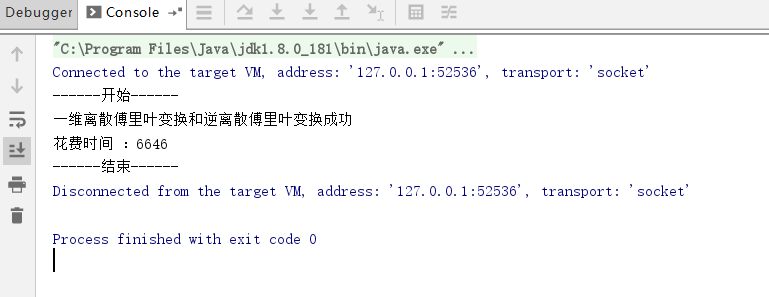展开全文IDFT 傅里叶变换
• ## 离散傅里叶变换

万次阅读 2018-01-21 08:21:13
傅里叶变换将信号分解为正弦波，离散傅里叶变换DFT基于数字信号。real DFT是将输入输出信号都用实数表示，一般用复数DFT，但实数DFT是基础。 傅里叶变换族 傅里叶变换是傅里叶在研究热传导时发现的，他提出用正弦...
傅里叶变换将信号分解为正弦波，离散傅里叶变换DFT基于数字信号。real DFT是将输入输出信号都用实数表示，一般用复数DFT，但实数DFT是基础。
傅里叶变换族
傅里叶变换是傅里叶在研究热传导时发现的，他提出用正弦波代表温度分布并向法兰西学会提交论文。但当时的法兰西学会权威拉格朗日对此理论并不赞成，拉格朗日认为傅里叶的方法不能代表非连续信号。实际上拉格朗日某些条件下是对的，因为正弦波之和确实无法表示非连续信号，但却可以无限接近，即两者能量无限接近。这种现象叫做吉布斯效应。当信号为离散信号时傅里叶分解完全成立，拉格朗日所拒绝的是连续信号。
一个16点长度信号被分解为正弦信号和余弦信号，如下图所示：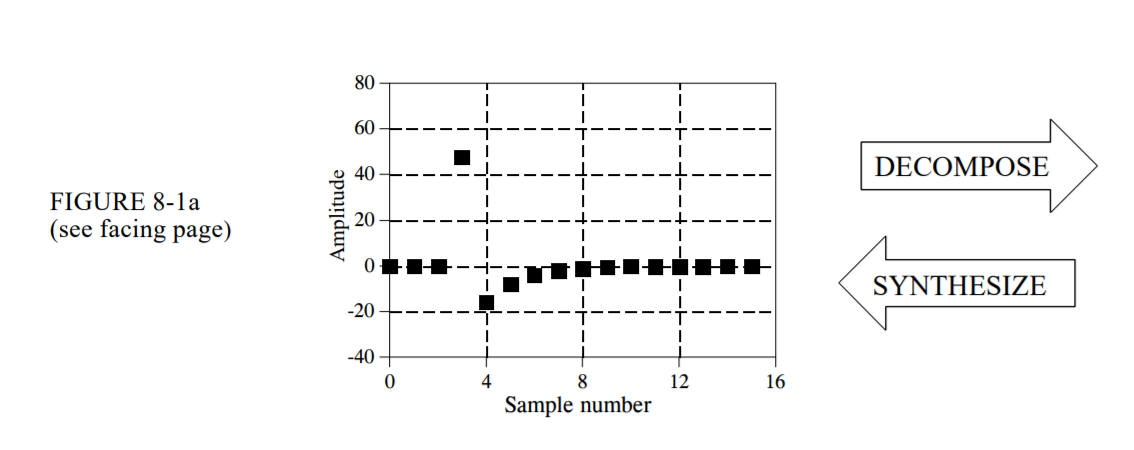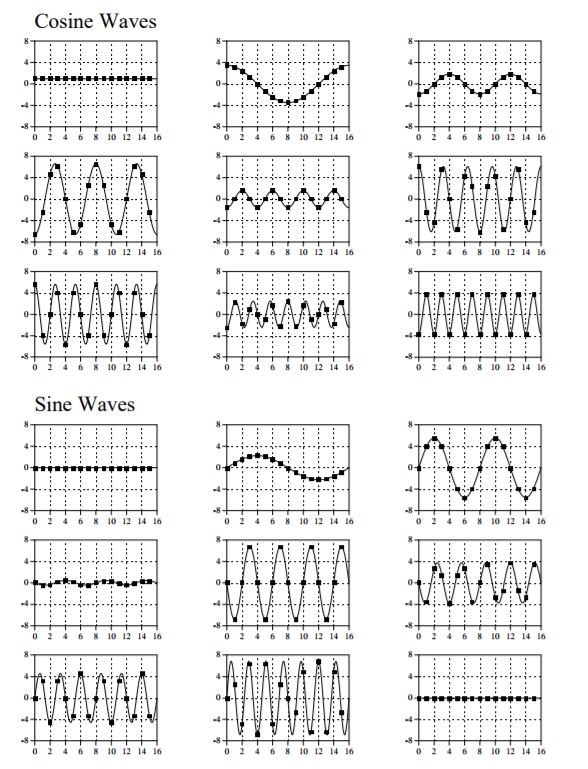如上图所示傅里叶分解将此信号分解为9个正弦信号和9个余弦信号。每个都有不同的幅度和频率。至于为何选用正弦波而不是三角波或者方波进行分解，这主要正弦信号特有的特性：正弦信号保真度。正弦信号输入到一个系统中其输出仍为正弦信号，其频率和波形保持不变，只有其幅度和相位发生改变。正弦曲线是唯一有此特性的波。
傅里叶变换可以根据4种不同信号分为4类，信号可以是离散或者连续的，也可能是周期的或者非周期的。因此可以分为以下4类：
非周期连续
这种信号傅里叶变换简单的叫做傅里叶变换FT
周期连续
这种信号傅里叶变换叫做傅里叶级数FS
非周期离散
这种信号傅里叶变换叫做离散时间傅里叶变换DTFT
周期离散
这种信号傅里叶变换叫做离散傅里叶变换DFT
这四种信号都延伸到正负无穷，是否有信号傅里叶变换可以转换有限长度信号？答案是没有，因为正弦波和余弦波都是延伸到无穷。你不能将一组无穷长度信号组合成一个有限长度信号。那处理有限长度信号时将其认为无线长度信号即可。如果将有限长度信号没有值的位置都认为是0则信号是离散非周期的，可以用DTFT；如果将没有值的位置认为是有信号位置处的简单复制，则信号可以认为是周期离散这时可以用DFT。实际上，对于非周期信号需要无限多个正弦波和余弦波来合成，因此实际在计算机算法中DTFT是不可能的。在实际应用中唯一可用的是DFT。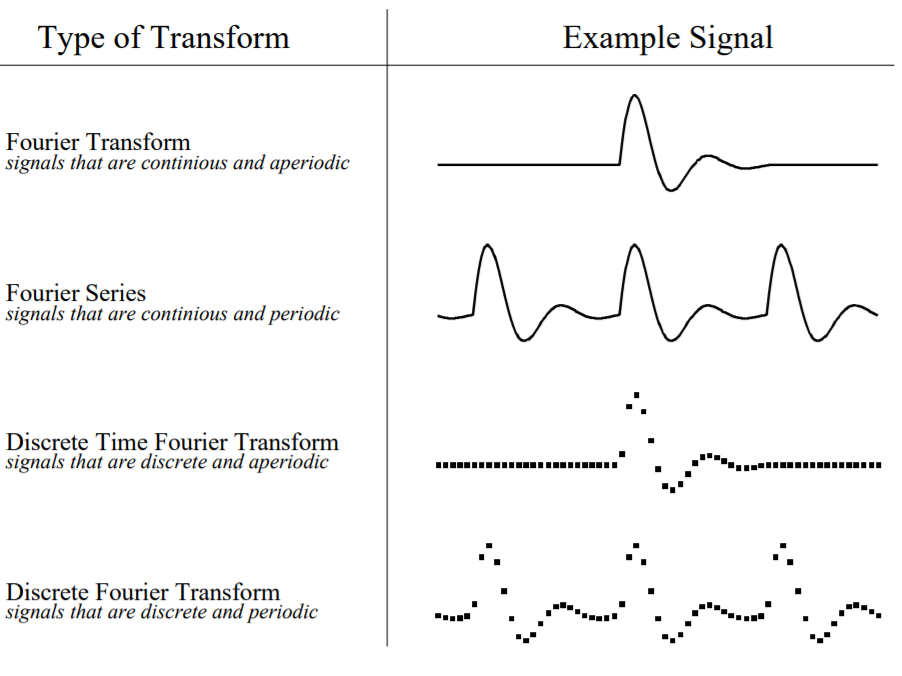对于第一个例子16个点的傅里叶变换，将其认为是16个点正弦波的合成的16个点的信号或者是由无限长正弦波合成的无限长周期信号是否有区别？一般来说没区别，但某些情况下会有所不同。
Real DFT
N点输入信号经过实数傅里叶变换转换为两个N/2+1点的输出信号。输入信号包含将要被分解的信号，两个输出信号包含正弦和余弦波分量的幅度。输入信号认为是时域信号因为一般是时域采样获得。频域用来描述正弦和余弦波的幅度。
频域和时域包含相同信息，只是不同的表现形式。给定一个域的信号就可以计算出另外一个。假如给定时域信号，计算频域信号的过程叫做分解、分析或者前向DFT。如果知道频域信号，计算时域信号叫做综合或者反向DFT。
频域中频率的表示
频域横坐标轴有4种不同表示方法：
1、0~N/2写程序者喜欢这一做法
2、分数采样率f范围0~0.5，因为离散数据可以包含DC到1/2采样率的频率。f=k/N。
3、自然频率w，将f乘以2π得到。数学家喜欢这种方法。
4、模拟频率，直观对应实际世界的意义。
DFT基函数
DFT中用到的正弦波和余弦波叫做DFT基函数。换句话说，DFT的输出是一组代表幅度的数。基函数是一组单位幅度的正弦波和余弦波。将这些正余弦波幅度设置为合适幅度将其相加就可以得到时域信号。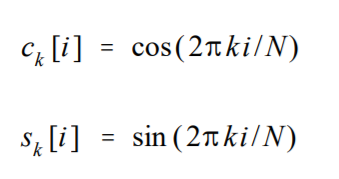如下图例子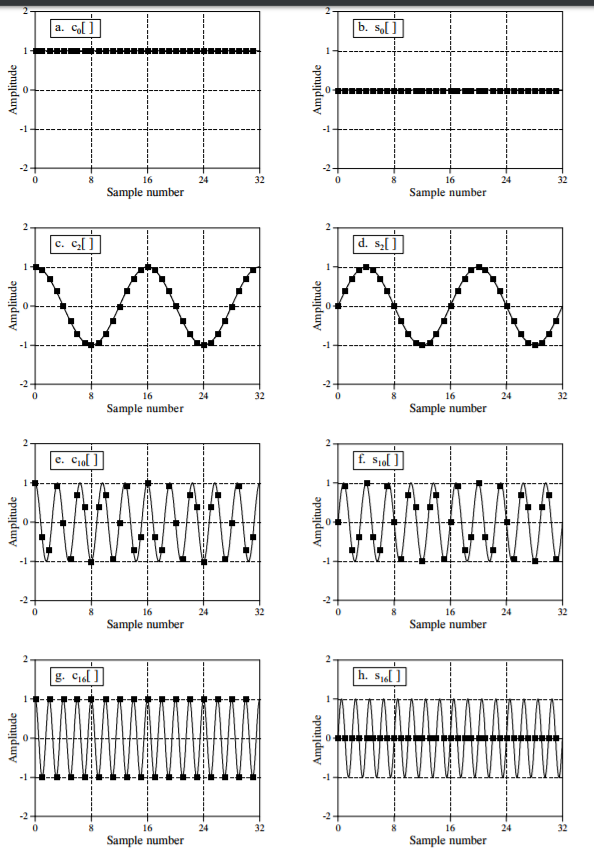c1[]是在N点中有一个完整周期的的余弦函数，c5[]是在N点中有5个完整周期的余弦函数。频率参数k等于N点信号的周期数。
如图c0[]值恒为1，他代表直流偏置DC offset。s0[]全零不影响时域信号。c16[]在+1和-1之间变换，采样连续正弦波的峰值。s16[]为全零。因此N点DFT输入，虽然输出有N+2个sample，但是有两个为全零即不包含任何信息。
综合，计算逆DFT（IDFT）
综合方程N点信号x[i]可以通过将N/2+1个余弦波和N/2+1个余弦波相加获得。正弦和余弦波的幅度乘以基函数并将这些相加就产生时域信号。注意综合所需的幅度与频域信号的幅度有些许不同。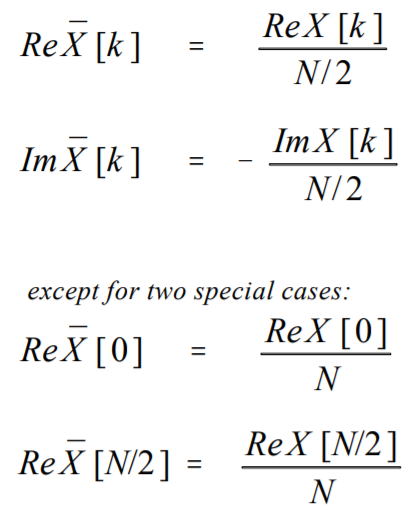下图给出了IDFT的例子，显示了频域幅度和与需要综合的幅度的差异，a是一个幅度为32的脉冲，b是a的频率响应，频率响应实部是恒定值32，虚部全为0。这是一个重要的DFT变换对，时域麦种对应于频域的恒定值。a和b是DFT变换对。将b中频域信号转换为需要综合的余弦波的幅度。
至于频域幅度和正弦波幅度为何有差异，那是因为频域根据谱密度定义。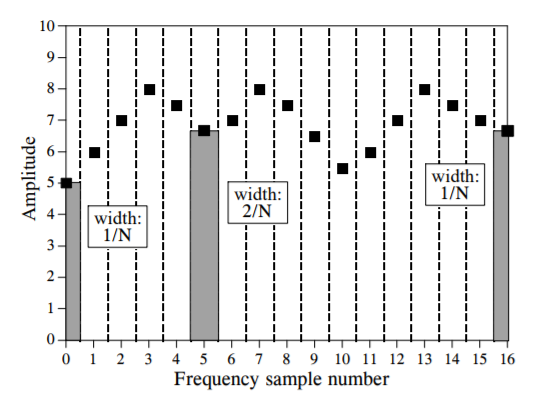分析，计算DFT
DFT可以根据三种完全不同的方法计算。第一种方法是联立方程法，这种方法对于理解DFT有益，但实际应用来说效率太低。第二种方法是相关，这种方法基于在另一种信号中检测已知的波形。第三种方法是快速傅里叶变换FFT。FFT算法将N点DFT转换为N个单点DFT。实际应用中DFT点数少于32点时用相关法，其他情况都用FFT法。
联立方程计算DFT
时域N个值计算频域N个值（忽略2个比为0的频域值），对于N个未知数需要N个线性独立方程。将每个正弦波第一个采样点相加得到时域第一个采样点也即第一个方程，同样方法可以总共得到N个方程。
相关法计算DFT
计算DFT的标准方法。假如我们想要计算64点的DFT，我们需要计算频域实部的33个点和虚部的33个点。假设对于虚部的ImX，表示要知道在0~63点之间三个完整周期正弦波的幅度。其他频域值的计算也类似。
下图阐述了用相关法计算ImX，上面两个显示了两个时域信号x1[]和x2[]，x1[]表示一个0-63点包含三个周期的正弦波，作为对比x2[]包括几个正弦和余弦波，这些组成中不包括0-63点有三个周期的正余弦波。这两个信号阐述了计算ImX的算法过程。对于x1[]，算法产生值32，也即正弦波代表的信号幅度。作为对比，对于x2[]，算法产生0，也意味着这个特殊的正弦波没在这个信号中出现。
相关的概念是将两个信号相乘并将所有点相加代表了一直波形在另一个信号的存在情况。这个过程的结果代表了两个信号的相似度。中间两个图显示了我们想要得到的信号即在采样点0~63有三个周期的正弦波。最下面图显示了两者的乘积。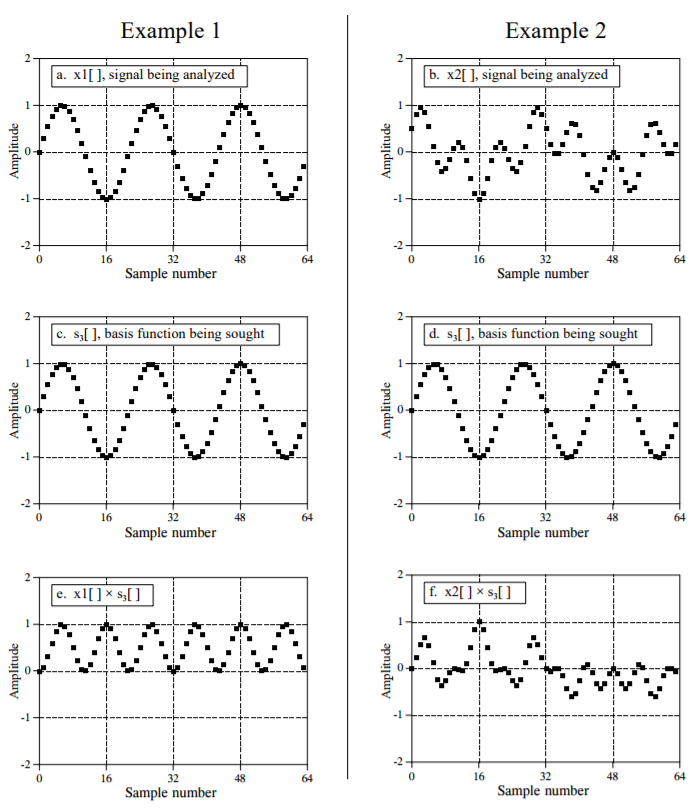频域其他采样点也用相同的方法计算。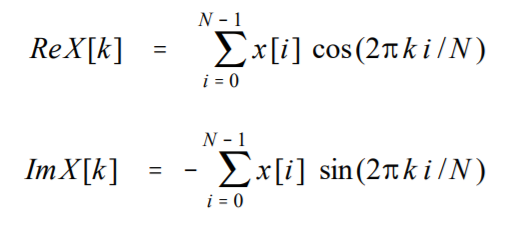分析方程不需要对第一个和最后一个点做特殊处理。但是对于虚部需要有个负号，负号是为了使实数DFT与复数DFT一致。为了使相关算法工作，基函数必须互不相关，也即正交。
二元性
综合和分析方程非常相似。为了从一个域转换到另一个域，将已知的值乘以基函数并且将结果相加。两个方程最大的区别是时域是N点信号，而频域是两个N/2+1点信号。而对于复数DFT时域频域都是包含N点。这样两个域就完全对称。二元性也带来一些有趣的性质，比如时域的一个点对应于频域的正弦波，反过来也成立即频域的一个点对应于时域的正弦波。另外一个例子是时域卷积对应于频域相乘，同时频域卷积对应于时域相乘。
极坐标表示
如前面描述频域是一组正余弦波的幅度表示，这种表示叫做矩形表示，频域也可以极坐标形式表示。即原来的实部和虚部表示可以转换为幅度和相位表示。实部和虚部与幅度和相位的样点是一一对应的。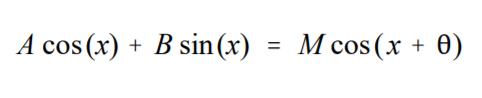矩形表示和极坐标表示是等价的不会损失任何信息。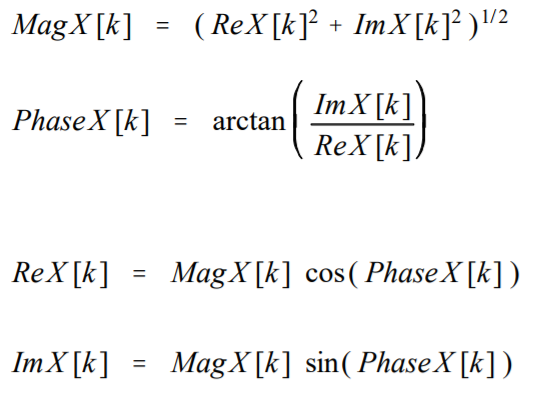矩形和极坐标表示可以让你用两种方法来考虑DFT。在矩形表示中DFT将N点信号分解为N/2+1个正弦波和N/2+1个余弦波，每一个有特定的幅度。在极坐标表示中，DFT将N点信号分解为N/2+1个余弦波形，每一个有特定的幅度和相移。对于极坐标表示为什么不用正弦波代替余弦波，因为正弦波不能表示直流分量DC。
尽管极坐标和矩形表示包含相同信息但很多例子表明一种表示会更好用。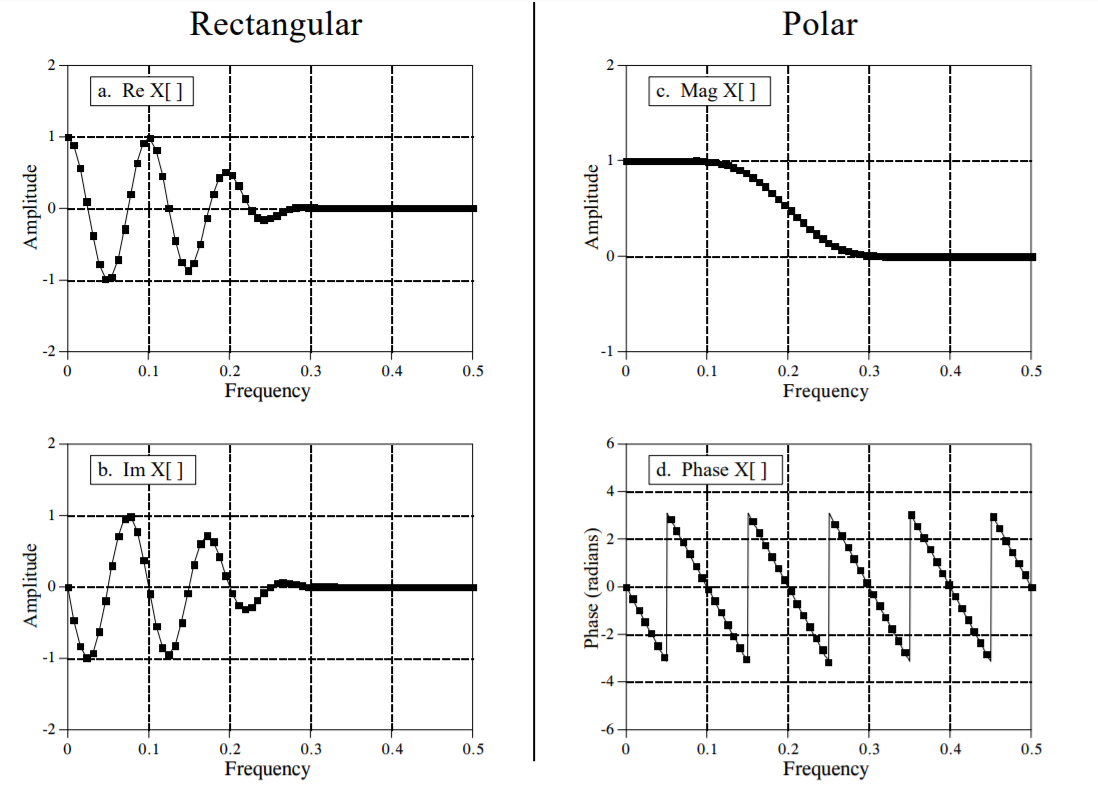矩形表示适合用来计算，作为对比图形表示一般用极坐标形式。为何频域表示更易懂？这也是为何将信号分解为正弦波形式有意义。如果将正弦波输入到线性系统，输出也将是同频率正弦波，只有幅度和相位发生改变。系统也可以用来表示他们如何改变每个正弦波的幅度和相位。而对于矩形表示，正弦波和余弦波进入线性系统，一个正弦波输入可能输出既有正弦波也有余弦波。
极坐标表示的缺点
弧度和度数
degree -180~180
除以0的错误
当从矩形形式转换到极坐标形式时，实部为0而虚部非0时相位为90度或者-90度，但是求arctan时除数为0.为避免这个问题，如果实部为0检查虚部的正负来确定相位。或者是将实部置为一个非常小的数来进行后续的除法。
不正确的arctan
ReX=1,ImX=1则Phase=45°，ReX=-1,ImX=-1则Phase=135°,但是直接求arctan时两者都为45°。实际计算完arctan后再根据实部和虚部的正负进行调整。
幅度非常小时的相位
幅度太小，舍入误差导致相位无意义。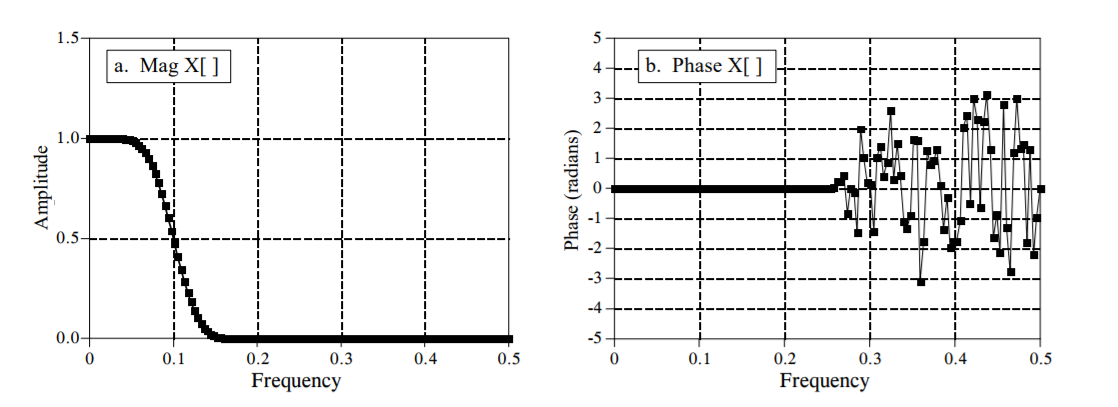2π相位模糊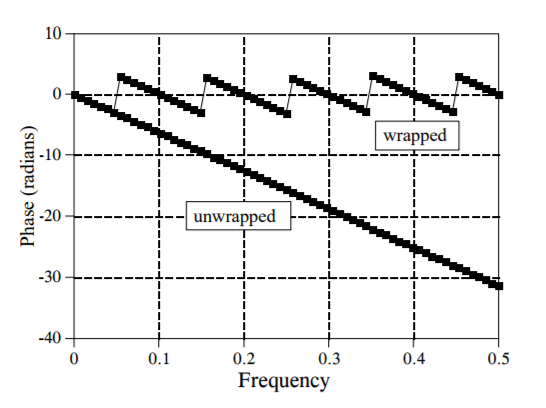幅度总为正（π相位模糊）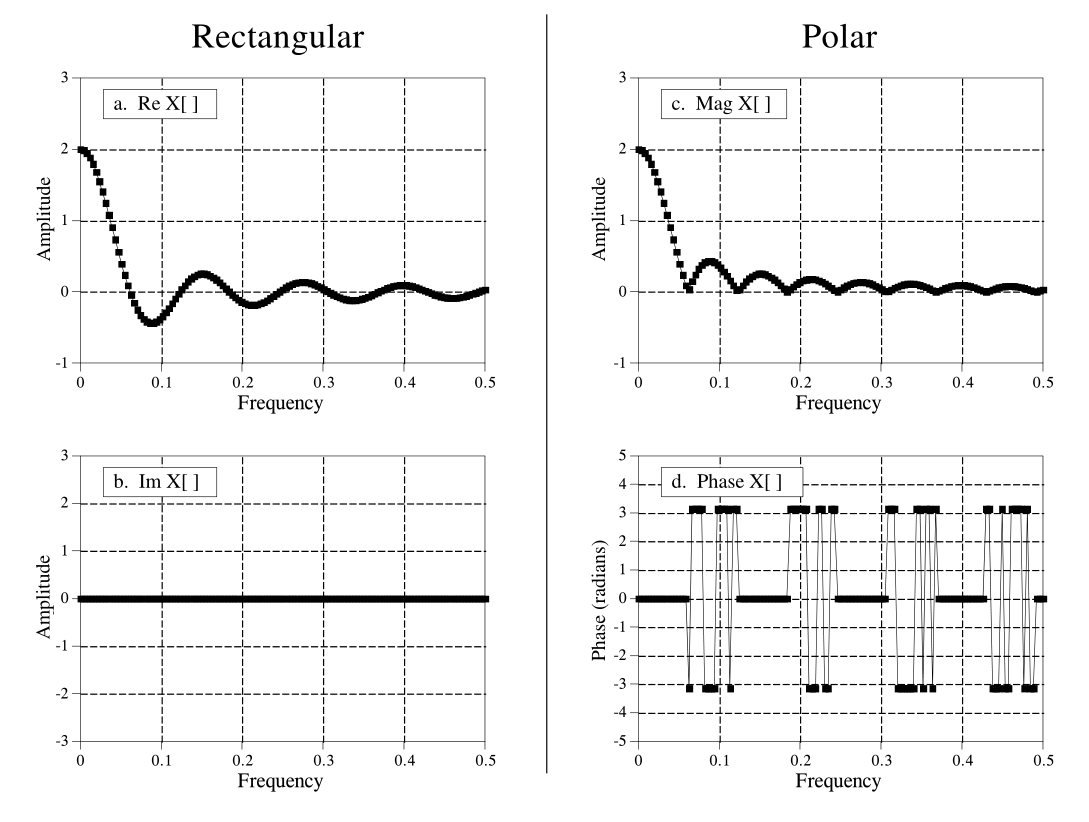幅度总为正值，当实部小于0时，幅度将相位改变π保持正值。一种方法是允许幅度有负值，这样幅度与实部相同值。相位为全0。用unwrapped 幅度表示允许有负值的幅度。


展开全文• N点离散傅里叶变换同时计算两个N点实序列的离散傅里叶变换matlab
• 实验二 应用快速离散傅里叶变换 (FFT)对信号进行频谱分析 一、实验目的 1、通过这一实验，能够熟练掌握快速离散傅里叶变换（FFT）的原理及其用FFT进行频谱分析的基本方法。 2、在通过计算机上用软件实现FFT及信号...FFT频谱分析
• ## 傅里叶变换(二维离散傅里叶变换)

万次阅读 多人点赞 2018-06-15 22:22:35
（1）可分离性： 二维离散傅里叶变换DFT可分离性的基本思想是DFT可分离为两次一维DFT。因此可以用通过计算两次一维的FFT来得到二维快速傅里叶FFT算法。根据快速傅里叶变换的计算要求，需要图像的行数、列数均满足2...
离散二维傅里叶变换一常用性质：       可分离性、周期性和共轭对称性、平移性、旋转性质、卷积与相关定理；（1）可分离性：   二维离散傅里叶变换DFT可分离性的基本思想是DFT可分离为两次一维DFT。因此可以用通过计算两次一维的FFT来得到二维快速傅里叶FFT算法。根据快速傅里叶变换的计算要求，需要图像的行数、列数均满足2的n次方，如果不满足，在计算FFT之前先要对图像补零以满足2的n次。   一个M行N列的二维图像f(x,y)，先按行队列变量y做一次长度为N的一维离散傅里叶变换，再将计算结果按列向对变量x做一次长度为M傅里叶变换就可以得到该图像的傅里叶变换结果，如式所示：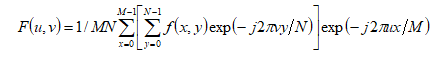将上式分解开来就是如下的两部分，先得到F(x,v),再由F(x,v)得到F(u,v)：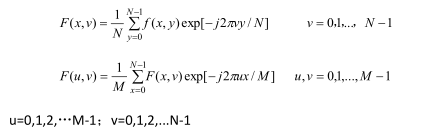计算过程如下：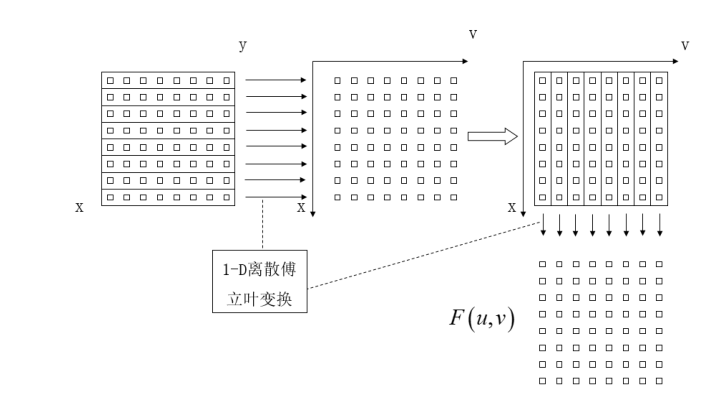每一行由N个点，对每一行的一维N点序列进行离散傅里叶变换得到F(x,u),再对得到F(x,u)按列向对每一列做M点的离散傅里叶变换，就可以得到二维图像f(x,y)的离散傅里叶变换F(u,v).同样，做傅里叶逆变换时，先对列向做一维傅里叶逆变换，再对行做一维逆傅里叶变换，如下式所示：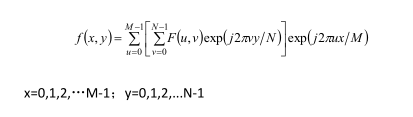（2）周期性和共轭对称性由傅里叶变换的基本性质可以知道，离散信号的频谱具有周期性。离散傅里叶变换DFT和它的里变换都以傅里叶变换的点数N为周期的。对于一维傅里叶变换有：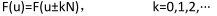对于二维傅里叶变换有：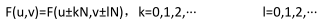类似有：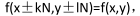即从DFT角度来看，反变换得到的图像阵列也是二维循环的。共轭对称性对于一维信号有：F(u)=F*(-u),如图所示的一维信号的幅度谱：点数为M的傅里叶变换一个周期为M，关于原点对称。原点即为0频率点，从图中可以看出在0频率的值最大，即信号f(x)的直流分量(均值)，远离原点处的即为高频成份，高频成份的幅值较小，说明信号的大部分能量集中在低频部分。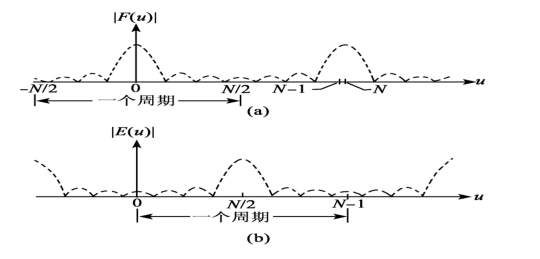对于二维信号有：F(u,v)=F*(-u,-v)对于二维图像，其结果如图c所示。左上角(0,0)处为二维图像得0频率点，该点得值对应图像的平均灰度值，图中四个角对应低频成分，中间区域为高频成份，低频区域的幅度值打羽高频区域的幅度值，也同样表示该信号的主要能量集中在低频区域。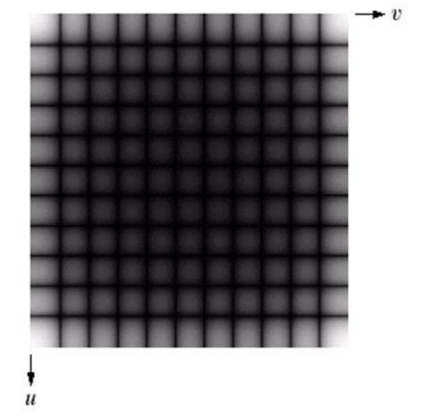根据周期性和共轭对称性，在对图像进行频谱分析处理时只需要关注一个周期就可以了，同时利用图像的傅里叶变换和傅里叶变换的共轭可以直接计算图像的幅度谱，因此使得图像的频谱计算和显示得以简化。（3)平移性：傅里叶变换对有如下平移性质：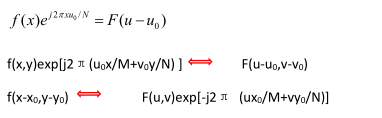式子表明，在频域中原点平移到(u0 ,v0)时，其对应的空间域 f(x,y)要乘上一个正的指数项：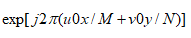在空域中图像原点平移到(x0,y0)时，其对应的F(u,v)要乘上一个负的指数项：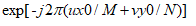在数字图像处理中，常常需要将F(u,v)的原点移到N*N频域的中心，以便能清楚地分析傅里叶谱的情况，平移前空域、频域原点均在左上方。要做到这点，只需令上面平移公式中的：u0=v0=N/2；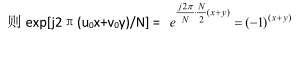所以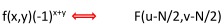上式表明：如果需要将图像傅里叶谱的原点从左上角(0,0)移到中心点(N/2,N/2),只要f(x,y)乘上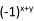因子进行傅里叶变换即可实现。平移性还体现了：当空域中f(x,y)产生移动时，在频域中只发生相移，并不影响他的傅里叶变换的幅度，因为：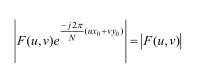反之，当频域中F(u,v)产生移动时，相应f(x,y)在空域中也只发生相移，不产生幅值变化。根据平移性质，为了更清楚查看二维图像的频谱，使直流成分出项在图像中央，在把画面分成四分的基础上，进行如图所示的换位(移位)也是可以的，这样，频域原点就回平移到中心。如下所示：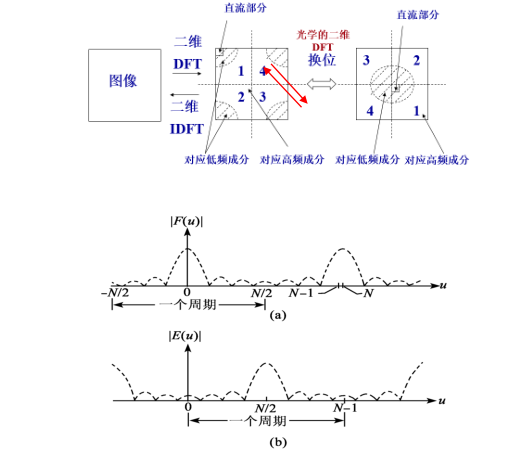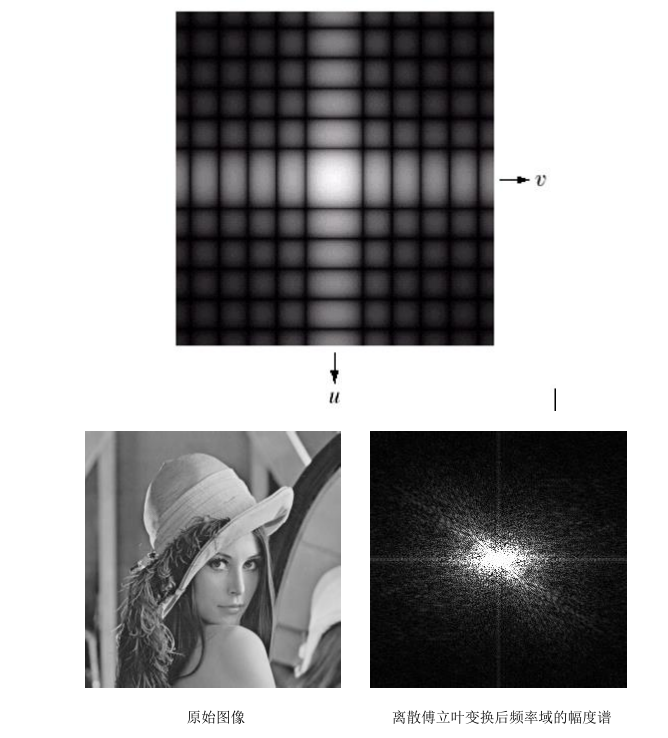（4）旋转性质如果 f(x,y)旋转了一个角度，那么 f(x,y)旋转后的图像的傅立叶变换也旋转了相同的角度。平面直角坐标改写成极坐标形式：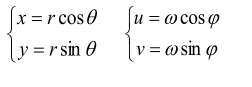替换则有：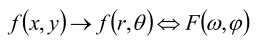如果f(x,y)被旋转W,则F(u,v)被旋转同一角度。即有傅里叶变换对：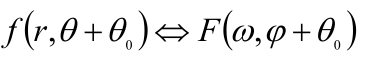如下所示：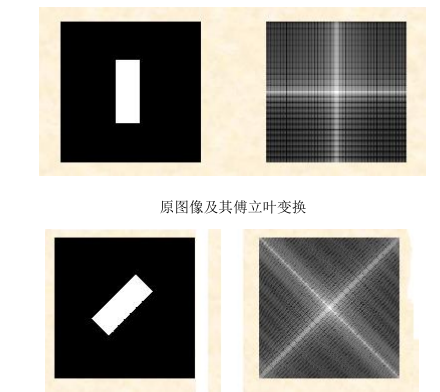同时，我们可以得出结论，对图像进行旋转变换和傅立叶变换的顺序是可交换的。即先旋转再傅里叶变换或者先傅里叶变换再旋转，得到的结果相同。F{R{f(x,y)}} = R{F{f(x,y)}}。（5）卷积与相关定理卷积定理包括空间域卷积和频率域卷积，卷积是空间域滤波和频率域滤波之间的纽带：两个空域信号的卷积等价于其频域信号的 乘积f(x,y)*h(x,y) → F(u,v)H(u,v) 或者 F{f(x,y)*h(x,y)} = F(u,v)H(u,v)；两个信号频域上的卷积等价于空间域的相乘f(x,y) g(x,y) →F(u,v)*H(u,v)；该性质的好处是将需要经过翻折、平移、相乘、求和等步骤实现的复杂的卷积运算简化为简单的乘法运算，这也是快速傅里叶变换（FFT）的出现使得该性质得到更广泛应用，同时，该性质对于理解信号的频率域处理方法特别重要，使得信号的空间域处理可以转换到频率域进行处理实现。根据空间域卷积定理，在空间域对应的是原始信号与滤波器的冲击响应的卷积，卷积定义式为信号翻折平移求和的过程，步骤复杂，运算量大，但如果转换到频率域进行处理，则对在将二者的频谱直接相乘就可以得到滤波结果，然后对滤波结果进行傅里叶逆变换就可以得到滤波后的空间域域图像。如下图所示，对信号进行低通和高通滤波处理的过程和效果。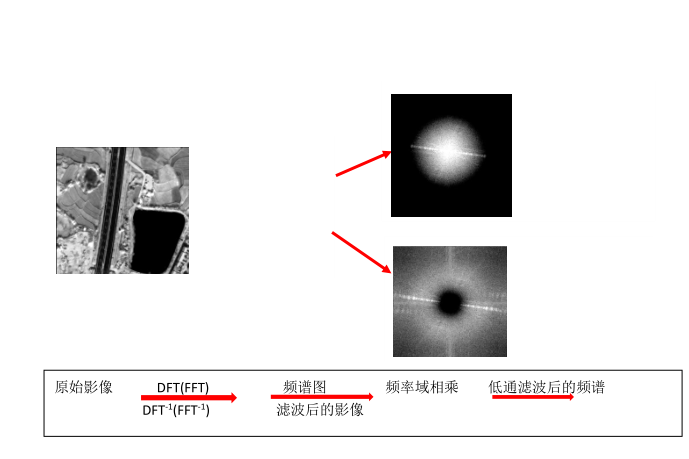相关定理：空域中 f(x,y)与 与 g(x,y) 的相关等价于频域中 F(u,v) 的共轭与 G(u,v)  相乘f(x,y) g(x,y) → F*(u,v)G(u,v)同时有：f*(x,y)g(x,y) → F(u,v) G(u,v)相关定理与卷积定理类似，也是把积分求和过程转化为了频域相乘，因此，也使得相关分析的计算简化。相关的重要应用在于匹配：确定是否有感兴趣的物体区域。f(x,y)是原始图像，g(x,y)作为感兴趣的物体或区域（模板），如果匹配，两个函数的相关值会在 f 中找到相应 g 点的位置上达到最大值。如下图所示。图像 f(x,y) 与模板 g(x,y)，通过计算相关函数，在匹配点处达到最大值，如图中红色圆圈标注的区域。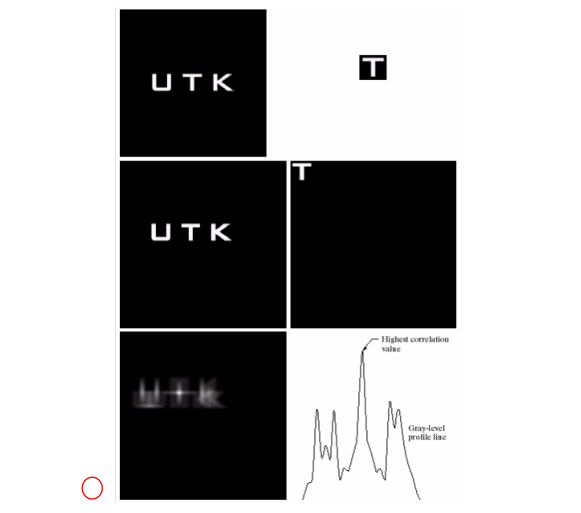延拓图像 f(x,y)，延拓图像 g(x,y)，相关函数图像，通过相关图像最大值的水平灰度剖面图。傅里叶变换的实例与应用首先我们认识几点有关傅里叶变换的特点：l 傅里叶变换是从将图像从空间域变换到频率域，具有明确的物理意义。图像的频率是表征图像中灰度变化剧烈程度的指标，是灰度在平面空间上的梯度，在噪声点和图像边缘处的频率为高频。l 在频率域中，将信号表示为一系列正弦信号或者复指数函数的叠加，正弦信号的频率、幅值和相位可以描述正弦信号中的所有信息，由此可以得到信号的幅度谱和相位谱。在图像领域就是将图像灰度作为正弦变量。l 傅里叶变换全局性的，是一个积分求和的过程，对时间、地点位置无法进行准确定义，也就是说傅里叶变换得到的频谱图中的点无法与空间域中的某个空间位置对应，因此，从傅里叶变换图中并不能直接对应某个位置的特点。l 傅里叶变换是一系列不同频率三角函数的和，每个频率分量的系数不同，这些系数代表了各频率成分的强弱或者所占比重，通过分析这些系数就可以分析图像的特性。
展开全文傅里叶变换性质
• 有限长的离散变换：离散傅里叶变换 Finite-Length Discrete Transforms： DFT(Discrete Fourier Transform) 1.正交变换（Orthogonal Transforms） 若对于基序列 ψ[k,n]，有： 那么对于有限长度的序列x[n]（0≤n......